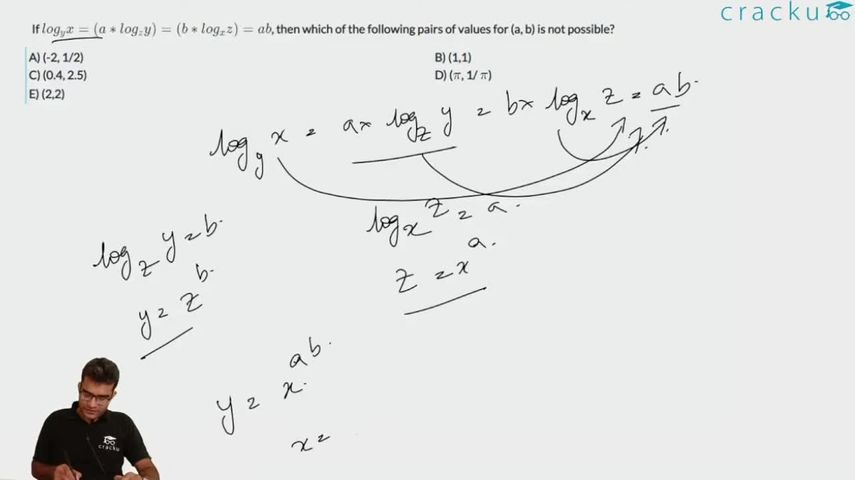Question 24

# If $$log_y x = (a*log_z y) = (b*log_x z) = ab$$, then which of the following pairs of values for (a, b) is not possible?

Solution

$$log_y x = ab$$
$$a*log_z y = ab$$ => $$log_z y = b$$
$$b*log_x z = ab$$ => $$log_x z = a$$
$$log_y x$$ = $$log_z y * log_x z$$ => $$log x/log y$$ = $$log y/log z * log z/log x$$
=> $$\frac{log x}{log y} = \frac{log y}{log x}$$
=> $$(log x)^2 = (log y)^2$$
=> $$log x = log y$$ or $$log x = -log y$$
So, x = y or x = 1/y
So, ab = 1 or -1
Option 5) is not possible

### View Video Solution